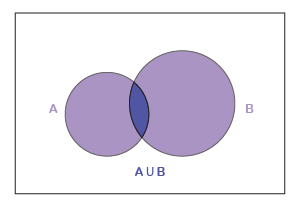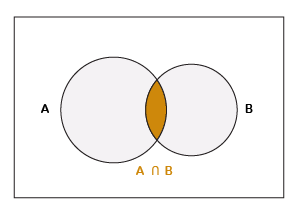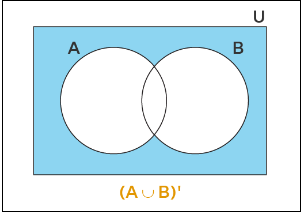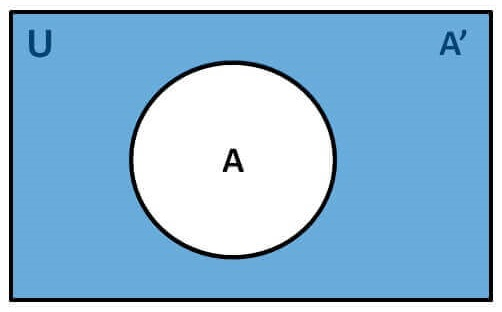Set theoretic operations using Venn diagrams

## Objective

To represent set theoretic operations using Venn diagrams

## Set & Set Operations

A set is a collection of well defined objects or groups of objects.

These objects are often called elements or members of a set. For example, a group of players in a cricket team is a set.

The symbols used while representing the operations of sets

• Union of sets symbol: ∪
• Intersection of sets symbol: ∩
• Complement of set: A’ or Ac
• Subset of  set: ⊂

### Operations for sets

• Union of sets : A∪B
• Intersection of Sets : A∩B
• Complement of Sets : A’
• Difference of Sets : A-B

## Venn Diagrams

Most of the relationships between sets can be represented by means of diagrams which are known as Venn diagrams.

These diagrams consist of rectangles and closed curves usually circles. The universal set is represented by rectangle and its subsets by circles.

A Venn diagram is a diagram that helps us visualize the logical relationship between sets and their elements and helps us solve examples based on these sets.

## Examples of different operations of sets using Venn Diagrams

A ∪ B : The union of two sets A and B is the set C which consists of all those elements which are either in A or in B (including those which are in both).

Symbolically we write A ∪ B = {x : x ∈A or x ∈B}

The union of two sets can be represented by a Venn diagram as shown in following figure.The shaded portion in figure represents A ∪ B.

Let A and B be any two sets. The union of A and B is the set which consists of all the elements of A and all the elements of B, the common elements being taken only once.

The symbol ‘∪’ is used to denote the union.

Symbolically, we write A ∪ B and usually read as ‘A union B’.

Example : Let A = {2, 4, 6, 8} and B = {6, 8, 10, 12}. Find A ∪ B.

Solution : We have A ∪ B = {2, 4, 6, 8, 10, 12}

A ∩ B The intersection of two sets A and B is the set of all those elements which belong to both A and B.

Symbolically, we write A ∩ B = {x : x ∈ A and x ∈ B}.

The shaded portion in Figure indicates the intersection of A and B.Example: Consider the sets A = {2, 4, 6, 8} and B = {6, 8, 10, 12}. Find A ∩ B.

Solution: We see that 6, 8 are the only elements which are common to both A and B.

A ∩ B = { 6, 8 }

#### (A ∪ B)' A union B Complement:  consists of those elements of the universal set U which are not in A U B.

The required formula can be written in any of the following forms: A Union B Complement is equal to the intersection of the complements of the two sets A and B.Example: Determine the elements of A union B complement if U = {1, 2, 3, 4, 5, 6, 7}, A = {2, 4, 6}, and B = {1, 3, 5}

Solution: We have A = {2, 4, 6}, and B = {1, 3, 5}, then A U B is given by, A U B = {1, 2, 3, 4, 5, 6}, then A union B complement is given by, (A ∪ B)' = U - (A ∪ B) = {1, 2, 3, 4, 5, 6, 7} - {1, 2, 3, 4, 5, 6} = {7}

Answer: (A ∪ B)' = {7}

#### (A ∩ B)' A Intersection B Complement consists of all elements of the universe except the elements in A Intersection B. A Intersection B Complement is equal to the union of the complements of the sets A and B.

Mathematically, it is written as (A ∩ B)' = A' U B'.

Example: Consider U = {1, 2, 3, 4, 5, 6, 7, 8, 9} and A' = {2, 3, 5} and B' = {1, 2, 3, 4, 5}. Find the elements in A Intersection B Complement.

Solution: We know that (A ∩ B)' = A' U B' Now, A' U B' = {2, 3, 5} U {1, 2, 3, 4, 5} = {1, 2, 3, 4, 5} ⇒ (A ∩ B)' = {1, 2, 3, 4, 5}

Answer:The elements in A Intersection B Complement are {1, 2, 3, 4, 5}.

#### A′ : If A is a subset of the universal set U, then its complement A′ is also a subset of U.

Let U be the universal set and A a subset of U. Then the complement of A is the set of all elements of U which are not the elements of A.

Symbolically, we write A′ to denote the complement of A with respect to U.

Thus, A′ = {x : x ∈ U and x ∉ A}.Obviously A′ = U – A. We note that the complement of a set A can be looked upon, alternatively, as the difference between a universal set U and the set A.

Let U be the universal set which consists of all prime numbers and A be the subset of U which consists of all those prime numbers that are not divisors of 42.

Thus, A = {x : x ∈ U and x is not a divisor of 42}. We see that 2 ∈ U but 2 ∉ A, because 2 is divisor of 42.

Similarly, 3 ∈ U but 3 ∉ A, and 7 ∈ U but 7 ∉ A. Now 2, 3 and 7 are the only elements of U which do not belong to A.

The set of these three prime numbers, i.e., the set {2, 3, 7} is called the Complement of A with respect to U, and is denoted by A′. So we have A′ = {2, 3, 7}.

Example: Let U = {1, 2, 3, 4, 5, 6, 7, 8, 9, 10} and A = {1, 3, 5, 7, 9}. Find A′.

Solution: We note that 2, 4, 6, 8, 10 are the only elements of U which do not belong to A. Answer : A′ = {2, 4, 6, 8,10}